# The NPAR1WAY Procedure

#### Simple Linear Rank Tests for Two-Sample Data

Statistics of the form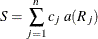are called simple linear rank statistics, where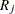is the rank of observation j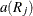is the score based on the rank of observation j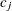is an indicator variable denoting the class to which the jth observation belongs

n

is the total number of observations

For two-sample data (where the observations are classified into two levels), PROC NPAR1WAY calculates simple linear rank statistics for the scores that you specify. The section Scores for Linear Rank and One-Way ANOVA Tests describes the available scores, which you can use to test for differences in location and differences in scale.

To compute the linear rank statistic S, PROC NPAR1WAY sums the scores of the observations in the smaller of the two samples. If both samples have the same number of observations, PROC NPAR1WAY sums those scores for the sample that appears first in the input data set.

For each score that you specify, PROC NPAR1WAY computes an asymptotic test of the null hypothesis of no difference between the two classification levels. Exact tests are also available for these two-sample linear rank statistics. PROC NPAR1WAY computes exact tests for each score type that you specify in the EXACT statement. See the section Exact Tests for details.

To compute an asymptotic test for a linear rank sum statistic, PROC NPAR1WAY uses a standardized test statistic z, which has an asymptotic standard normal distribution under the null hypothesis. The standardized test statistic is computed as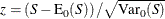where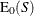is the expected value of S under the null hypothesis, and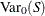is the variance under the null hypothesis. As shown in Randles and Wolfe (1979),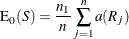where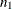is the number of observations in the first (smaller) class level (sample),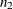is the number of observations in the other class level, and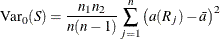whereis the average score,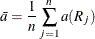##### Definition of p-Values

PROC NPAR1WAY computes one-sided and two-sided asymptotic p-values for each two-sample linear rank test. When the test statistic z is greater than its null hypothesis expected value of zero, PROC NPAR1WAY computes the right-sided p-value, which is the probability of a larger value of the statistic occurring under the null hypothesis. When the test statistic is less than or equal to zero, PROC NPAR1WAY computes the left-sided p-value, which is the probability of a smaller value of the statistic occurring under the null hypothesis. The one-sided p-value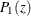can be expressed as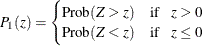where Z has a standard normal distribution. The two-sided p-value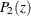is computed as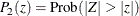##### Continuity Correction

PROC NPAR1WAY uses a continuity correction for the asymptotic two-sample Wilcoxon and Siegel-Tukey tests by default. You can remove the continuity correction by specifying the CORRECT=NO option. PROC NPAR1WAY incorporates the continuity correction when computing the standardized test statistic z by subtracting 0.5 from the numerator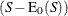if it is greater than zero. If the numerator is less than zero, PROC NPAR1WAY adds 0.5. Some sources recommend a continuity correction for nonparametric tests that use a continuous distribution to approximate a discrete distribution. (See Sheskin 1997.)

If you specify CORRECT=NO, PROC NPAR1WAY does not use a continuity correction for any test.